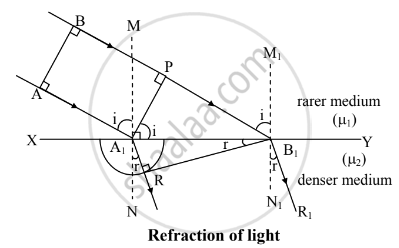# On the Basis of Huygens' Wave Theory of Light Prove That Velocity of Light in a Rarer Medium is Greater than Velocity Of Light in a Denser Medium. - Physics

On the basis of Huygens' wave theory of light prove that velocity of light in a rarer medium is greater than velocity of light in a denser medium.

#### SolutionXY : plane refracting surface

AB : incident plane wavefront

B1R : refracted wavefront

AA1 , BB1 : incident rays

A1R , B1R1 : refracted rays

∠ AA1M = ∠ BB1M1 = ∠ i : angle of incidence

∠ RA1N = ∠ R1B1N1 = ∠ r : angle of refraction

1.  Let XY be the plane refracting surface separating two media rarer and denser of refractive indices μ1 and μ2 respectively.
2. A plane wavefront AB is advancing obliquely towards XY from rarer medium. It is bounded by rays AA1 and BB1 which are incident rays.
3. When 'A' reaches at 'A1' then 'B' will be at 'P' . It still has to cover distance PB1 to reach XY.
4. According to Huygens' principle , secondary wavelets will originate from A1 and it will spread over a hemisphere in denser medium.
5. All the rays between AA1 and BB1 will reach XY and spread over the hemispheres of increasing radii in denser medium. The surface of tangency of all such hemisphere is RB1 . This gives rise to refracted wavefront B1R in denser medium.
6. A1R and B1R are refracted rays.
7. Let c1 and c2 be the velocities of light in rarer and denser medium respectively.
8. At any instant of time 't' , distance covered by incident wavelength from P to B1 = PB1 = c1t
Distance covered by secondary wave from A1  to R = A1R =c2t .
9. From above figure ,
∠ AA1M + ∠ MA1P = 90°      .....(i)  and
∠ MA1P + ∠ PA1B1 = 90°    ...........(ii)
From equation (i) and (ii) , we have,
∠ AA1M = ∠ PA1B1 = 90°
10. Similarly ,
∠NA1R = ∠ N1B1R1 = r
We have,
∠ N1B1R1 + ∠ A1B1R =  90°    .........(iii)
and
∠ N1B1R1 + ∠ A1B1R = 90°     .........(iv)
From equations (iii) and (iv) , we have,
∠ N1B1R1 +  ∠ A1B1R = r
11. In Δ A1PB1 ,
sin i = "PB"_1/("A"_1"B"_1) = ("c"_1"t")/("A"_1"B"_1)   ........(v)
12. In Δ A1RB1 ,
sin r = ("A"_1"R")/("A"_1"B"_1) = ("c"_2"t")/("A"_1"B"_1)        ............(vi)
13. Dividing equation (v) bt (vi), we have
("sin" "i")/("sin""r") = ((c_1t) / (A_1B_1))/((c_2t)/(A_1B_1))
therefore ("sin" "i")/("sin""r") = c_1/c_2  .....(vii)
Also c_1/c_2 = mu_2/mu_1 = 1 mu_2 .....(viii)
where 1μ2 = R.I. of denser medium w.r.t rarer medium.
14. From above figure ,
∠ i > ∠ r
∴ sin i > sin r
∴ ("sin" "i")/("sin" "r") > 1
therefore mu_2/mu_1 > 1   .........(ix)
Since , c_1/c_2 = mu_2/mu_1    ..........[From ix]
therefore c_1/c_2 > 1
therefore c_1 > c_2
Hence , velocity of light in rarer medium is greater than veocity in denser medium.
Concept: Huygens Principle
Is there an error in this question or solution?